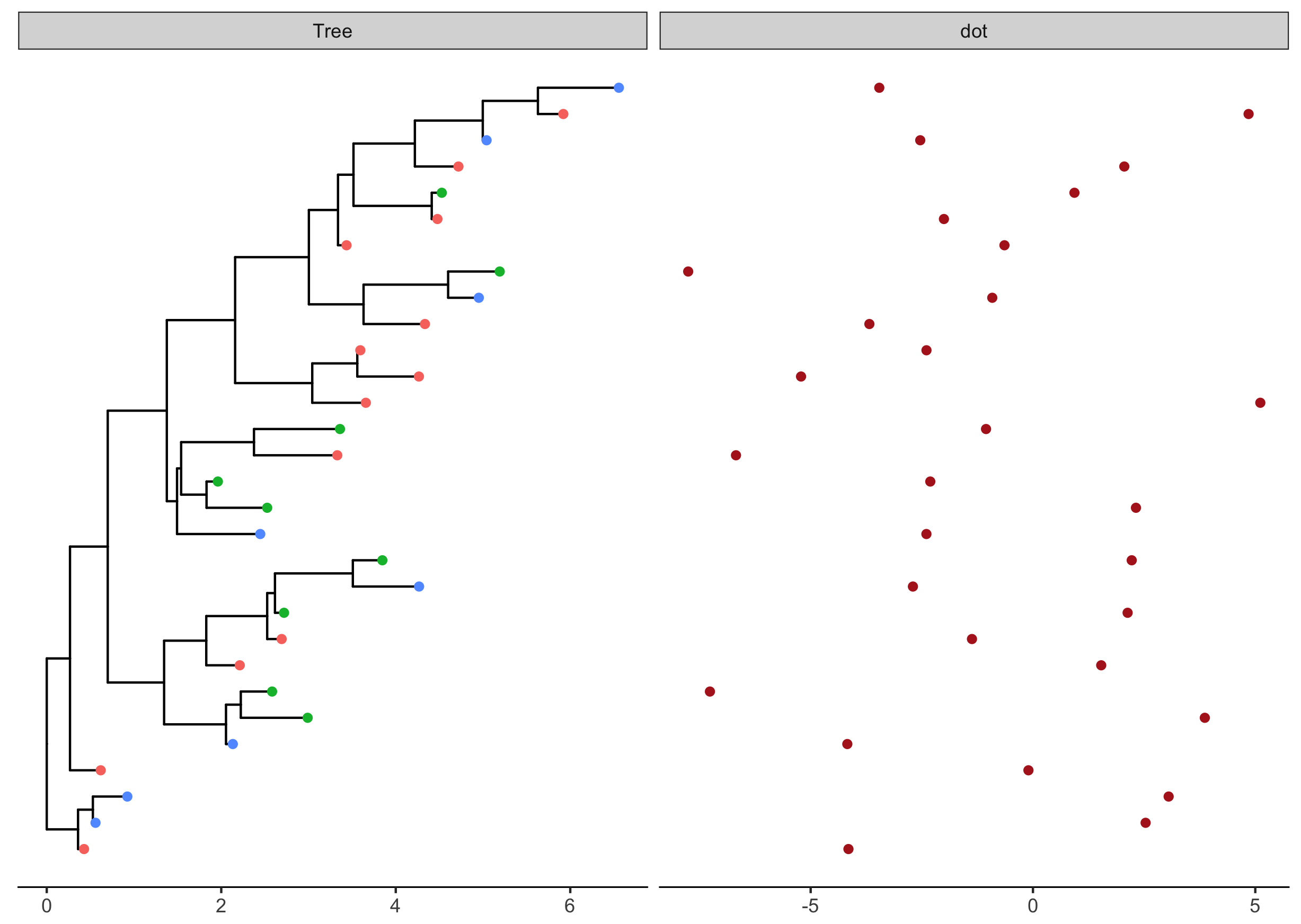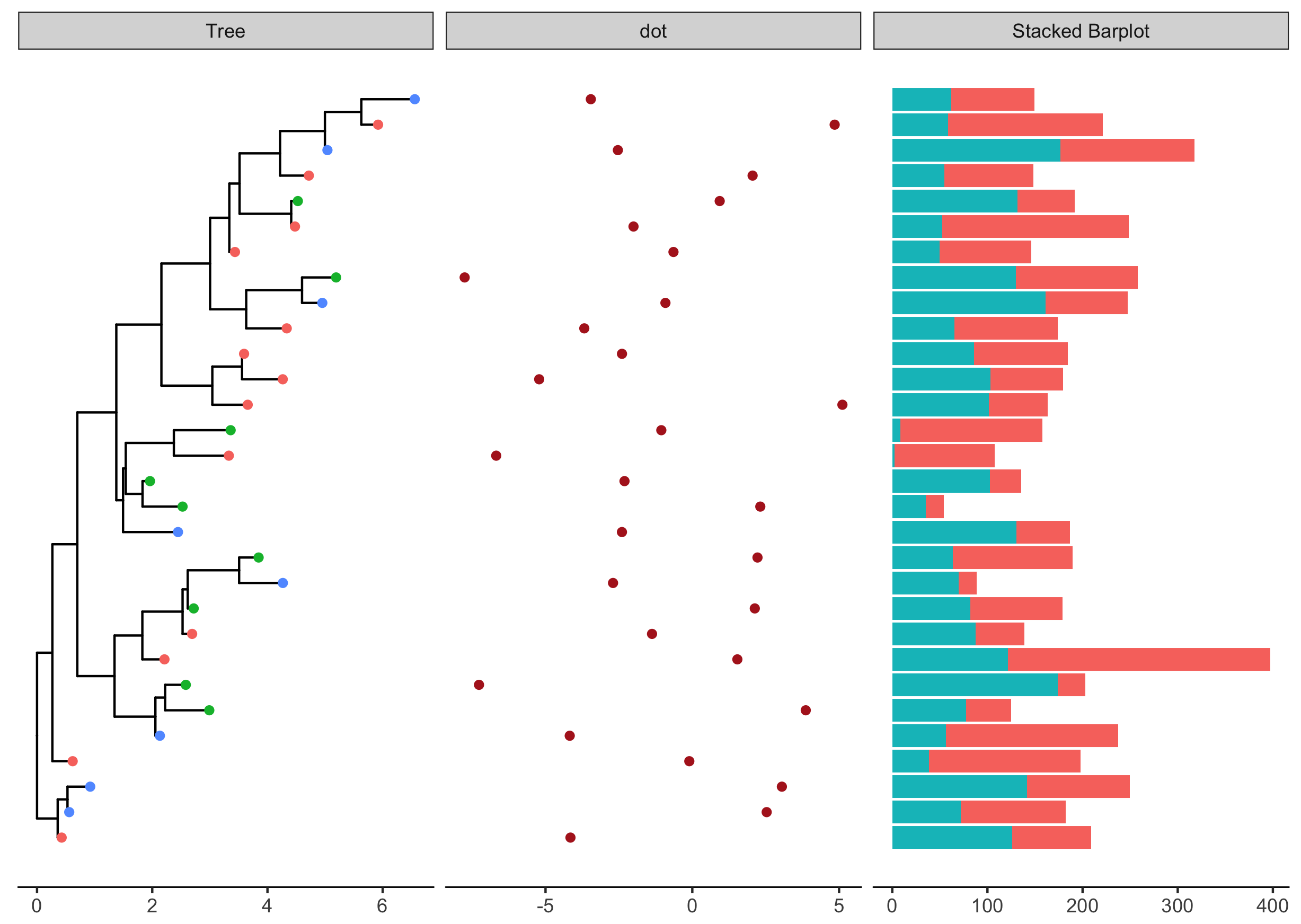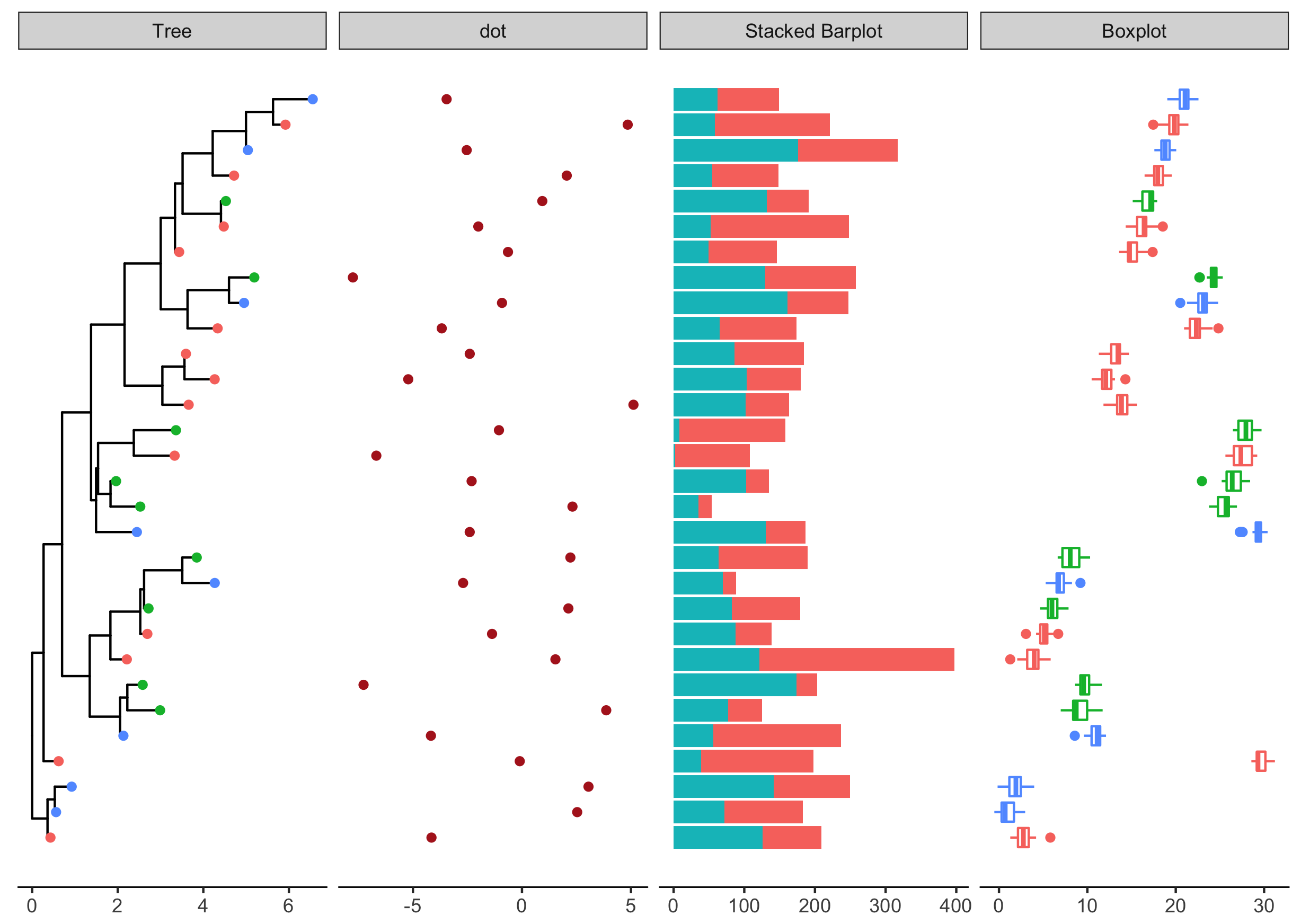ggtree provides `gheatmap` for visualizing heatmap and `msaplot` for visualizing multiple sequence alignment with phylogenetic tree.

We may have different data types and want to visualize and align them with the tree. For example, `dotplot` of SNP site (e.g. using `geom_point(shape='|')`), `barplot` of trait values (e.g. using `geom_barh(stat='identity')`) et al.

To make it easy to associate different types of data with phylogenetic tree, I implemented the `facet_plot` function which accepts a `geom` function to draw the input `data.frame` and display it in an additional `panel`.tr <- rtree(30) p <- ggtree(tr) d1 <- data.frame(id=tr\$tip.label, location=sample(c(“GZ”, “HK”, “CZ”), 30, replace=TRUE)) p1 <- p %<+% d1 + geom_tippoint(aes(color=location)) d2 <- data.frame(id=tr\$tip.label, val=rnorm(30, sd=3)) p2 <- facet_plot(p1, panel=“dot”, data=d2, geom=geom_point, aes(x=val), color=‘firebrick’) + theme_tree2()Most of the `geom` in `ggplot2` draw vertical graph object, while for associating graph object with phylogenetic tree, we need horizontal versions. Luckily, we have ggstance which provides horizontal versions of `geoms`, including:

• `geom_barh()`
• `geom_histogramh()`
• `geom_linerangeh()`
• `geom_pointrangeh()`
• `geom_errorbarh()`
• `geom_crossbarh()`
• `geom_boxploth()`
• `geom_violinh()`

With `ggstance`, we can associate `barplot`, `boxplot` or other graphs to phylogenetic trees.

``````library(ggstance)
d3 <- data.frame(id = rep(tr\$tip.label, each=2),
value = abs(rnorm(60, mean=100, sd=50)),
category = rep(LETTERS[1:2], 30))
p3 <- facet_plot(p2, panel = 'Stacked Barplot', data = d3,
geom = geom_barh,
mapping = aes(x = value, fill = as.factor(category)),
stat='identity' )
````````````d4 = data.frame(id=rep(tr\$tip.label, each=20),
val=as.vector(sapply(1:30, function(i)
rnorm(20, mean=i)))
)
p4 <- facet_plot(p3, panel="Boxplot", data=d4, geom_boxploth,
mapping = aes(x=val, group=label, color=location))
``````## Citation

G Yu, DK Smith, H Zhu, Y Guan, TTY Lam*. ggtree: an R package for visualization and annotation of phylogenetic trees with their covariates and other associated data. Methods in Ecology and Evolution. `doi:10.1111/2041-210X.12628`.